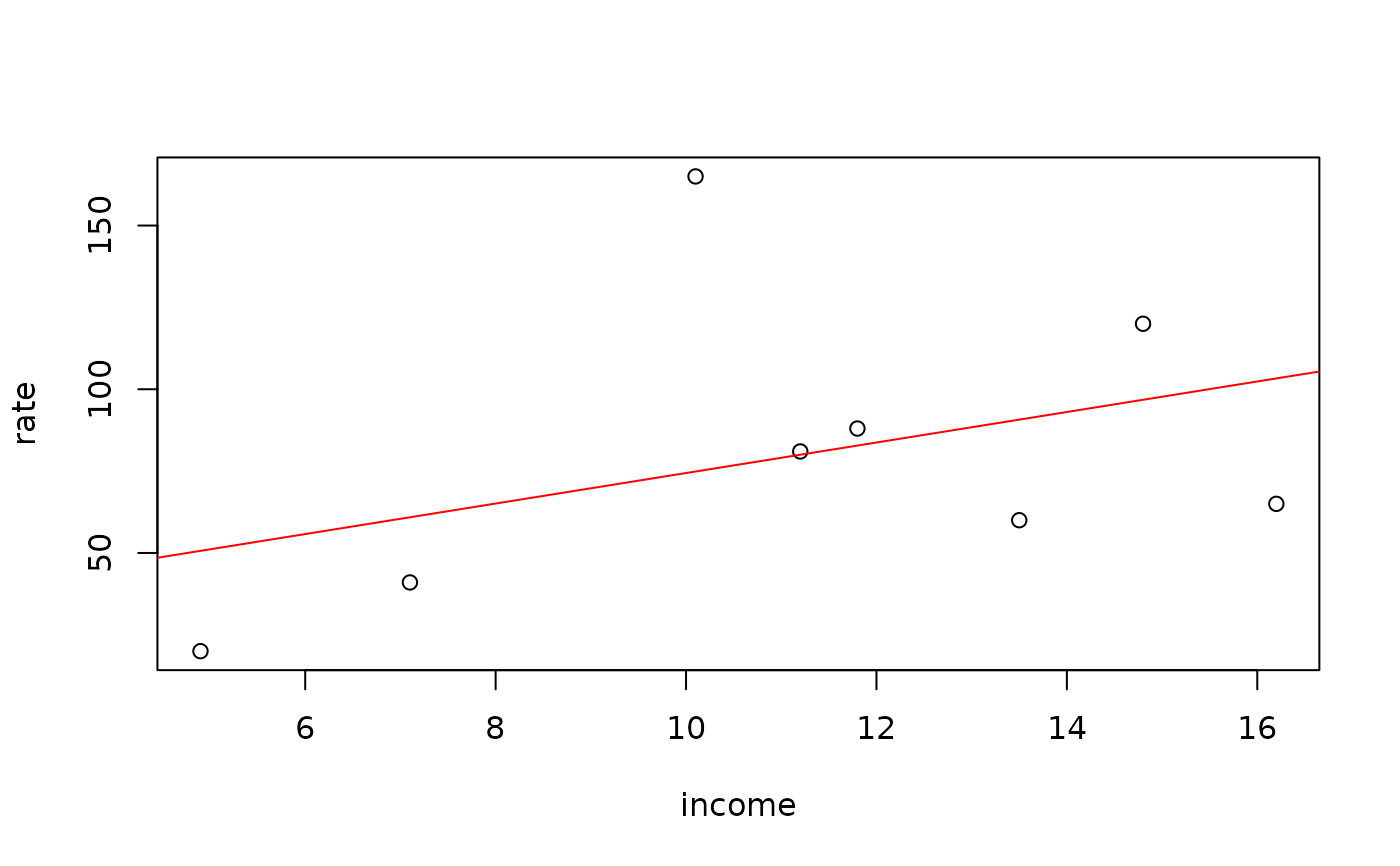Data for Exercise 2.2 and 2.38

Precinct

## Format

A data frame/tibble with eight observations on two variables

rate

robbery rate (per 1000 people)

income

percent with low income

## Examples


plot(rate ~ income, data = Precinct)
model <- (lm(rate ~ income, data = Precinct))
abline(model, col = "red")rm(model)